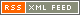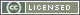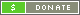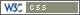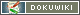# Fractional Dividers in a PLD

From the Time Nuts mailing list:

A 10 MHz clock into a PIC gives a 400 ns/instruction time.

To produce 32.768 kHz you flip an output pin put every 38
instructions, except that 9632 times per second you make it 39

To figure out those sorts of ratios for static frequency dividers, Allan Herriman has written a nifty fractional divider script that generates VHDL/Verilog code for CPLD/FPGA applications:

It will generate dual modulus or phase accumulator based dividers, with various implementation options.

To generate a 10 Mhz to 32.768 Khz with a frequency error tolerance of 1e-12 :

fracn09.pl -t 1e-12 10e6 32768

Which generates a file “fracn.vhd”:

```--
-- Frequency Results (use_phase_accumulator = FALSE) :
--  Achieved Output Frequency: 32768 Hz.
--  Achieved Relative Frequency Error: 0 (0 ppm)
--  Achieved Frequency Error: 0 Hz.
--```
```constant n              : positive := 305;  -- prescaler divides by n or n + 1
constant a              : positive := 211;  -- this many counts of 305
constant b              : natural  := 45;   -- this many counts of 306
```
```--
-- Design Parameters (use_phase_accumulator = FALSE) :
--  Approx 20 flip flops (9 in prescaler, 9 in controller and 2 retimes).
--  The recursive controller uses approx 17 flip flops.
--  The Dual-Modulus Prescaler uses ratios /305,/306
--  The Output consists of 211 cycles of 305 input clocks,
--  and 45 cycles of 306 input clocks.
--  There are 256 output clocks for every 78125 input clocks.
--```

Brian